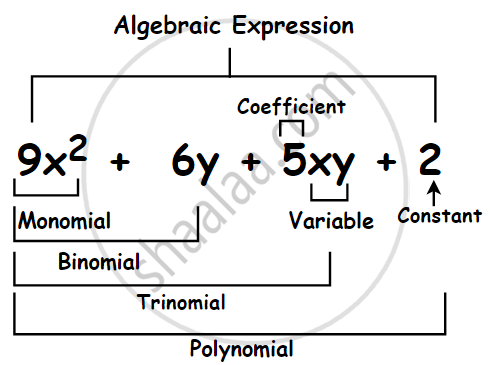# Types of Algebraic Expressions as Monomials, Binomials, Trinomials, and Polynomials:## 1. Monomial:

• Expression that contains only one term is called a monomial.
• Examples of monomials: 4x2, 3xy, –7z, 5xy2, 10y, –9, 82 mnp, etc.

## 2. Binomials:

• Expression that contains two terms is called a binomial.
• Examples of binomials: a + b, 4l + 5m, a + 4, 5 – 3xy, z2 – 4y2, etc.

## 3. Trinomial:

• An expression containing three terms is a trinomial.
• Examples of trinomials: a + b + c, 2x + 3y – 5, x2y – xy2 + y2, etc.

## 4. Polynomials:

• In general, any expression containing one or more terms with non-zero coefficients (and with variables having non- negative integers as exponents) is called a polynomial.
• Examples of polynomials: a + b+ c + d, 3xy, 7xyz – 10, 2x + 3y + 7z, etc.
If you would like to contribute notes or other learning material, please submit them using the button below.

### Shaalaa.com

Monomials, Binomials, Trinomials & Polynomials [00:15:38]
S
0%